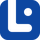# cardinal numbers

### Cardinal Numbers

When we want to refer to quantity, we use numbers. These numbers are called 'cardinal numbers.'

### Numbers

If math describes how the world works, numbers are the alphabet that makes math possible. In this lesson, we will discover all about them.

### Fractions, Decimals and Percentages

A fraction is a particular form of writing numbers. In this lesson, we will learn how to use them.

### Numbers

If math describes how the world works, numbers are the alphabet that makes math possible. In this lesson, we will discover all about them.

### Ordinal Numbers

Imagine that you are on a list and you want to know where you are standing. To refer to that number, we use 'ordinal numbers.'

### Expressing Time

Expressing time is not just about time and numbers. In this lesson, we will learn how to tell time and learn more about it.

### Ordinal Numbers

Imagine that you are on a list and you want to know where you are standing. To refer to that number, we use 'ordinal numbers.'

### Expressing Dates

Telling the date is one of the most common subjects in our daily life. In this lesson, we will learn how to express the date of something.

### Money & Prices

Talking about money and prices is an important part of every day language. Here we can learn how to talk about money and prices.